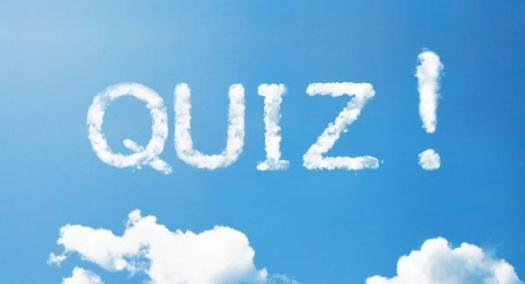# Dr. Mishari Alfraih -- Quiz # 5

8 QuestionsSettingsAccounting 203 -- Quiz # 5

• 1.
The financial statement that reports assets, liabilities, and owner's equity is the:
• A.

Income statement

• B.

Owner’s equity statement

• C.

Balance sheet

• D.

Statement of cash flow

• 2.
The financial statement that reports revenues and expenses is the:
• A.

Income statement

• B.

Owner’s equity statement

• C.

Balance sheet

• D.

Statement of cash flow

• 3.
The left side of an account is called:
• A.

Debit

• B.

Journal

• C.

Credit

• D.

Asset

• 4.
The right side of an account is called:
• A.

Debit

• B.

Journal

• C.

Credit

• D.

Asset

• 5.
The basic accounting equation is Assets = Liabilities +
• A.

Revenues

• B.

Owner’s equity

• C.

Expenses

• D.

Owner’s drawings

• 6.
Assets - liabilities =
• A.

Expenses

• B.

Owner's equity

• C.

Revenues

• D.

Cash

• 7.
Liabilities + Owner's equity =
• A.

Expenses

• B.

Assets

• C.

Revenues

• D.

Cash

• 8.
Which of the following will cause owner's equity to increase?
• A.

Expenses

• B.

Owner’s drawings

• C.

Revenues

• D.

Loss